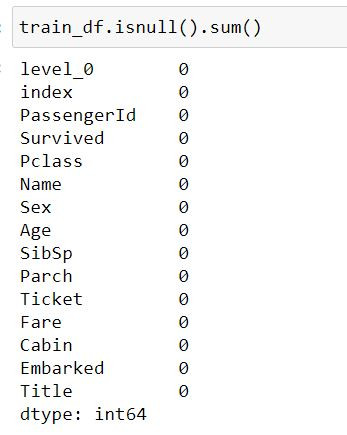DAY 8
1
AI & Data

一、缺失值(Missing Value)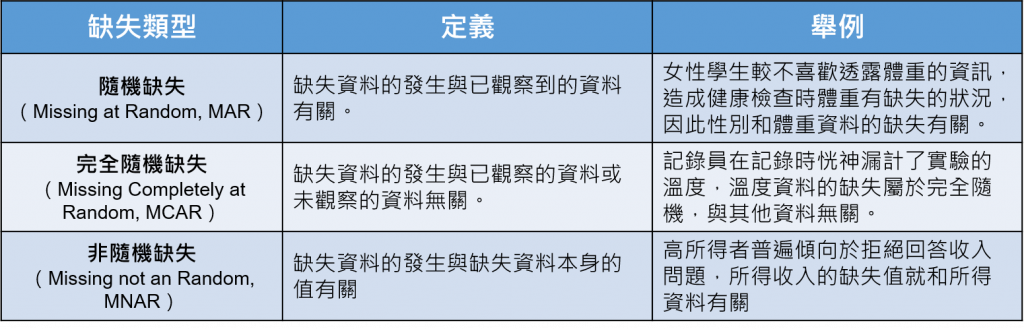二、缺失值處理方法

▲優點：

▲缺點：

-補值

▲以一個固定值去填補，例如全部補0

▲依照時間順序去補值(跟時間序列有關的資料)

▲依照現有資料的平均值、中位數、眾數...等去補值

▲透過機器學習的預測方法去補值

三、範例(鐵達尼號生存預測)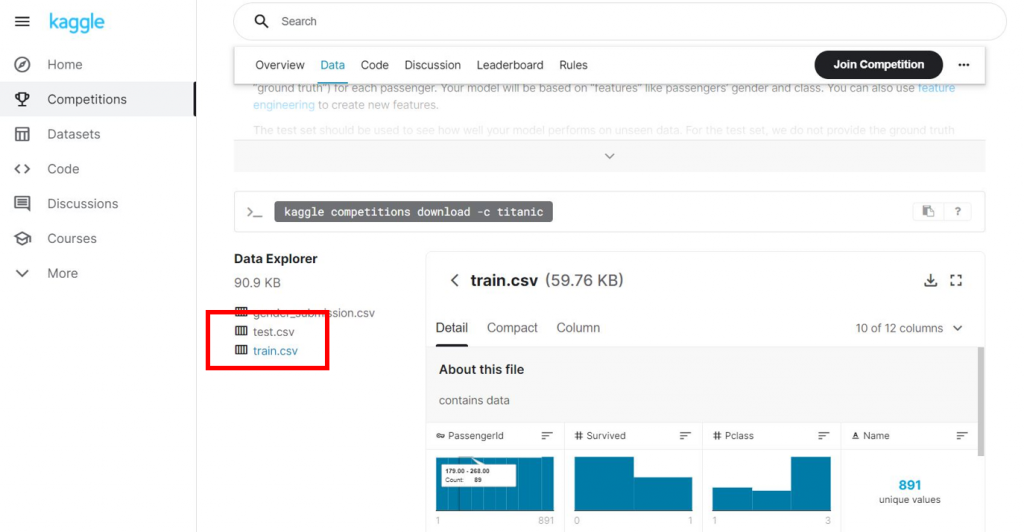下載完畢以後開啟Jupyter Notebook並將資料集上傳至環境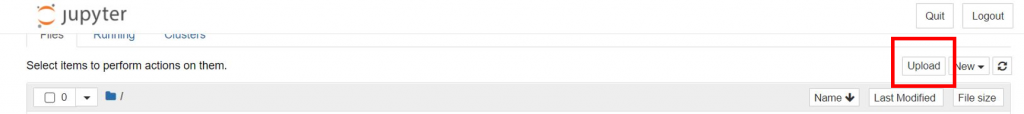import我們需要用到的套件

import pandas as pd
import numpy as np

可以看到這筆資料共有891筆資料，11種特徵+是否生還的標籤(0、1)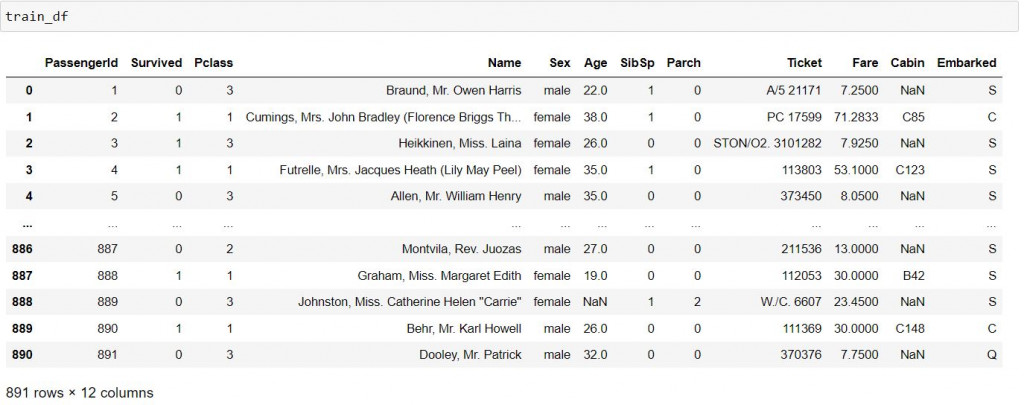幫大家整理成表格比較好理解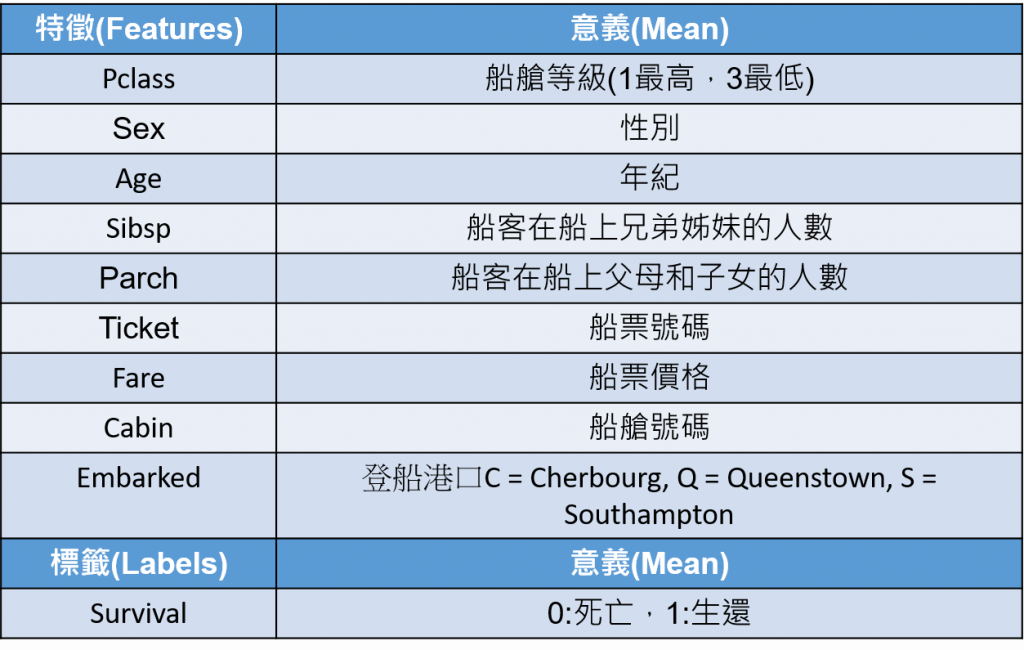找出含有缺失值的特徵

train_df.insull().sum()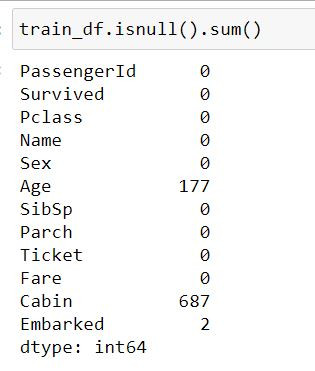這裡也給大家一個能夠視覺化成表格的def

def Missing_Counts( Data, NoMissing=True ) :
missing = Data.isnull().sum()

if NoMissing==False :
missing = missing[ missing>0 ]

missing.sort_values( ascending=False, inplace=True )
Missing_Count = pd.DataFrame( { 'Column Name':missing.index, 'Missing Count':missing.values } )
Missing_Count[ 'Percentage(%)' ] = Missing_Count['Missing Count'].apply( lambda x: '{:.2%}'.format(x/Data.shape ))
return  Missing_Count

Missing_Counts(train_df)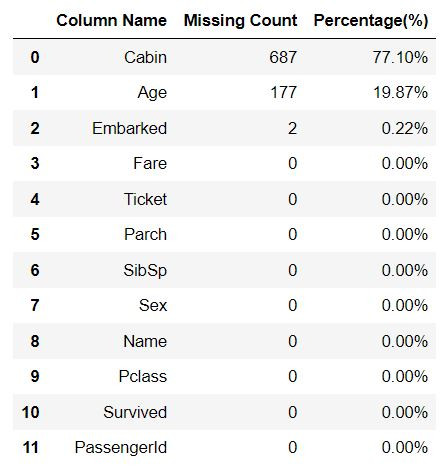首先我們可以看到Embarked的缺失數量非常少，只有2筆而已，我們可以選擇簡單填補或刪除，那這邊示範刪除的方法，其實非常簡單，只要一行程式即可。

train_df=train_df.dropna(subset=["Embarked"]) #subset參數裡面放要刪除缺失值的特徵

查看Cabin的資料類型分布

train_df['Cabin'].unique()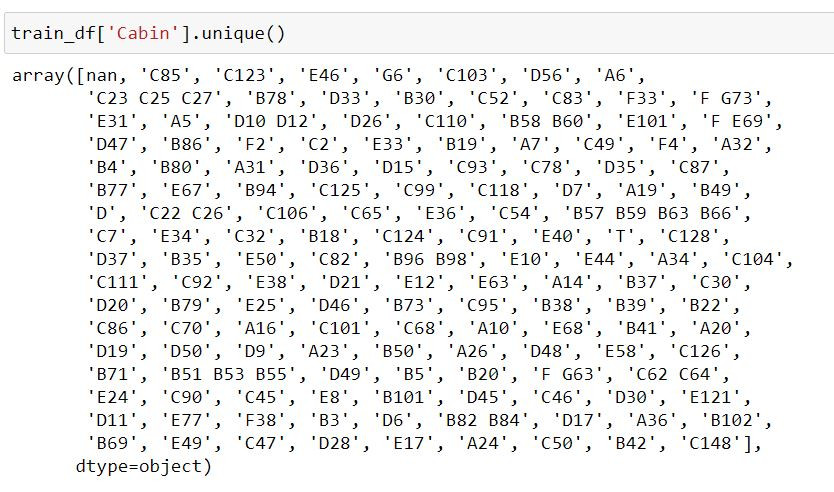train_df['Cabin']=train_df['Cabin'].fillna("No_Cabin")

利用箱形圖查看Age與Sex的關係觀察缺失資料在船艙等級(Pclass)的分布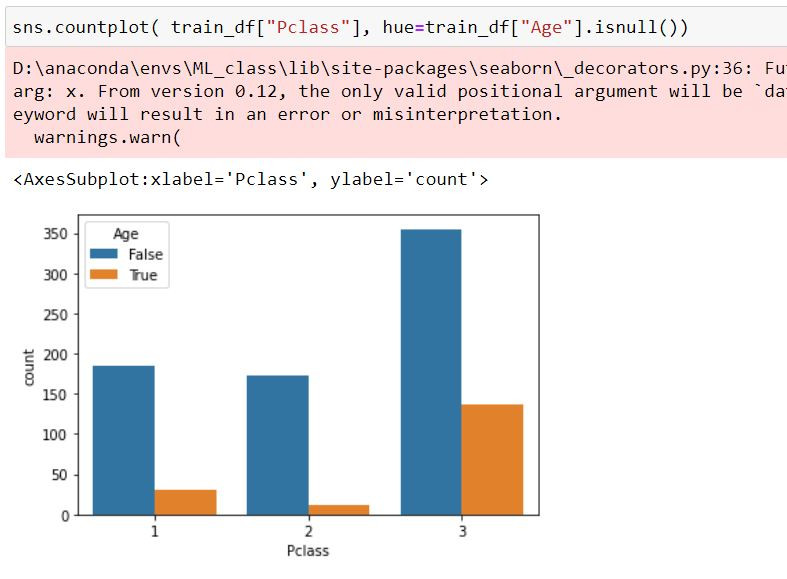觀察Age和Survived的相關性

index_survived = (train_df["Age"].isnull()==False)&(train_df["Survived"]==1)
index_died = (train_df["Age"].isnull()==False)&(train_df["Survived"]==0)

sns.distplot( train_df.loc[index_survived ,'Age'], bins=20, color='blue', label='Survived' )
sns.distplot( train_df.loc[index_died ,'Age'], bins=20, color='red', label='Survived' )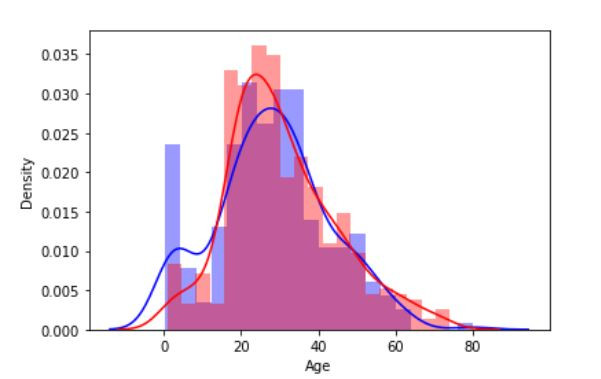Age和Name的相關性

train_df['Title'] = train_df.Name.str.split(', ', expand=True)
train_df['Title'] = train_df.Title.str.split('.', expand=True)
train_df['Title'].unique()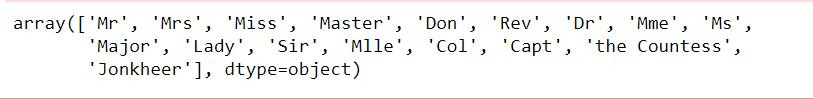計算每個Title的年齡平均

# 計算每個 Title 的年齡平均值
Age_Mean = train_df[['Title','Age']].groupby( by=['Title'] ).mean()

Age_Mean.columns = ['Age_Mean']
Age_Mean.reset_index( inplace=True )

display( Age_Mean )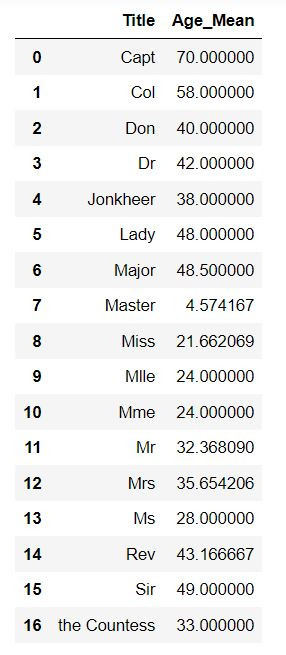根據缺失值的Title所對應的平均進行補值

train_df=train_df.reset_index() #重整index
train_df["Age"].isnull()
for i in range(len(train_df["Age"].isnull())):
if train_df["Age"].isnull()[i]==True:
for j in range(len(Age_Mean.Title)):
if train_df["Title"][i]==Age_Mean.Title[j]:
train_df["Age"][i]=Age_Mean.Age_Mean[j]

這樣一來缺失值就填補完畢啦，最後我們確定一下